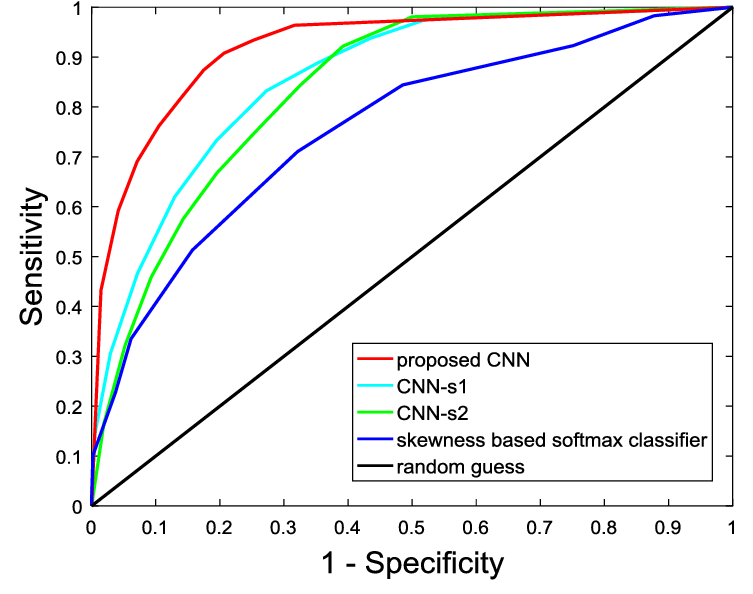Prince Home Stay Follish, Stay Hungry

# 目标检测任务中mAP的计算

• 参考博客 http://blog.sina.com.cn/s/blog_9db078090102whzw.html

### 多标签分类中的AUC与mAP（图片来自网络）

#### 一个简单的例子

index 1 2 3 4 5 6 7
confidence 0.9 0.8 0.7 0.5 0.3 0.2 0.1
GT 1 0 1 1 0 0 0
cumsum 1 1 2 3 3 3 3
Recall 1/3 1/3 2/3 3/3 3/3 3/3 3/3
Precision 1/1 1/2 2/3 3/4 3/5 3/6 3/7

• TP = cumsum(GT)
• Recall = TP / sum(GT)
• Precision = TP / index

### 检测中的mAP

• TP: 预测框与某个GT的IoU大于t，且没有更高置信度的预测框覆盖该GT
• FP: 预测框与所有的GT的IoU都小于t，或是与某个GT的IoU大于t，但是该GT已经被置信度跟高的预测框所覆盖
• TN: ？？
• FN: 与任何预测框的IoU都小于阈值t的GT数量

### VOC2007检测指标

#### 11-point interpolated average precision

confidence 0.9 0.8 0.7 0.6 0.6 0.5 0.4 0.3 0.2 0.1
TP 1 1 0 1 1 1 1 0 0 0
FP 0 0 1 0 0 0 0 1 1 1
TP_cumsum 1 2 2 3 4 5 6 6 6 6
FP_cumsum 0 0 1 1 1 1 1 2 3 4

• VOC2007: 为了计算速度，VOC2007的指标只在横轴上采样11个，分别是(0.0, 0.1, 0.2, 0.3, 0.4, 0.5, 0.6, 0.7, 0.8, 0.9, 1.0)，在这些点上求precision，加和以后除以11，也就是所谓的11-point interpolated average precision，这里有一个问题，0.1这个点上不一定有我们算出来的recall的点，所以voc07的指标选用了recall>0.1的所有precision中最大的precision作为0.1的precision，是一个估算
• VOC2012: VOC2012采用了更加合理的计算方式，不按照固定点采样，而是就使用每个坐标点对的的recall作为横坐标采样(r1, p1)(r2, p2)… (rn, pn)，类似直方图计算面积一样，计算出每个长方形的距离，最后相加除以recall的总长度就是AP了

#### Python版VOC指标代码分析

def voc_eval(detpath,
annopath,
imagesetfile,
classname,
cachedir,
ovthresh=0.5,
use_07_metric=False):

# 首先获取GT路径
if not os.path.isdir(cachedir):
os.mkdir(cachedir)
cachefile = os.path.join(cachedir, '%s_annots.pkl' % imagesetfile)
# 读取图片的ID
with open(imagesetfile, 'r') as f:
lines = f.readlines()
imagenames = [x.strip() for x in lines]

# 载入GT的标注，如果cache已经存在，直接读取，否则读文件，写cache
if not os.path.isfile(cachefile):
# load annotations
recs = {}
for i, imagename in enumerate(imagenames):
recs[imagename] = parse_rec(annopath.format(imagename))
if i % 100 == 0:
print('Reading annotation for {:d}/{:d}'.format(
i + 1, len(imagenames)))
# save
print('Saving cached annotations to {:s}'.format(cachefile))
with open(cachefile, 'wb') as f:
pickle.dump(recs, f)
else:
# load
with open(cachefile, 'rb') as f:
try:
recs = pickle.load(f)
except:
recs = pickle.load(f, encoding='bytes')

# extract gt objects for this class
class_recs = {}
npos = 0
for imagename in imagenames:
R = [obj for obj in recs[imagename] if obj['name'] == classname]
bbox = np.array([x['bbox'] for x in R])
difficult = np.array([x['difficult'] for x in R]).astype(np.bool)
det = [False] * len(R)
npos = npos + sum(~difficult)
class_recs[imagename] = {'bbox': bbox,
'difficult': difficult,
'det': det}

# 加载模型预测框
# 格式[img_id, x1, y1, x2, y2]共5维
detfile = detpath.format(classname)
with open(detfile, 'r') as f:
lines = f.readlines()

splitlines = [x.strip().split(' ') for x in lines]
image_ids = [x for x in splitlines]
confidence = np.array([float(x) for x in splitlines])
BB = np.array([[float(z) for z in x[2:]] for x in splitlines])

nd = len(image_ids) # 注意这里是预测框的总数量，而不是图片的数量
tp = np.zeros(nd) # 初始化每个框的TP标志
fp = np.zeros(nd) # 初始化每个框的FP标志

if BB.shape > 0:
# sort by confidence
sorted_ind = np.argsort(-confidence)
sorted_scores = np.sort(-confidence)
BB = BB[sorted_ind, :]
image_ids = [image_ids[x] for x in sorted_ind]

# go down dets and mark TPs and FPs
for d in range(nd):
R = class_recs[image_ids[d]]
bb = BB[d, :].astype(float)
ovmax = -np.inf
BBGT = R['bbox'].astype(float)

if BBGT.size > 0:
# compute overlaps
# intersection
ixmin = np.maximum(BBGT[:, 0], bb)
iymin = np.maximum(BBGT[:, 1], bb)
ixmax = np.minimum(BBGT[:, 2], bb)
iymax = np.minimum(BBGT[:, 3], bb)
iw = np.maximum(ixmax - ixmin + 1., 0.)
ih = np.maximum(iymax - iymin + 1., 0.)
inters = iw * ih

# union
uni = ((bb - bb + 1.) * (bb - bb + 1.) +
(BBGT[:, 2] - BBGT[:, 0] + 1.) *
(BBGT[:, 3] - BBGT[:, 1] + 1.) - inters)

overlaps = inters / uni
ovmax = np.max(overlaps)
jmax = np.argmax(overlaps)

if ovmax > ovthresh:
if not R['difficult'][jmax]:
if not R['det'][jmax]:
tp[d] = 1.
R['det'][jmax] = 1
else:
fp[d] = 1.
else:
fp[d] = 1.

# compute precision recall
fp = np.cumsum(fp)  # 对FP和TP求cumsum
tp = np.cumsum(tp)  # TP_cumsum + FP_cumsum其实就等于当前预测框的综述，也就是P
rec = tp / float(npos)
# avoid divide by zero in case the first detection matches a difficult
# ground truth
prec = tp / np.maximum(tp + fp, np.finfo(np.float64).eps)
ap = voc_ap(rec, prec, use_07_metric)

return rec, prec, ap

def voc_ap(rec, prec, use_07_metric=False):
""" ap = voc_ap(rec, prec, [use_07_metric])
Compute VOC AP given precision and recall.
If use_07_metric is true, uses the
VOC 07 11 point method (default:False).
"""
if use_07_metric: # VOC07采用11点采样的方法计算线下面积
# 11 point metric
ap = 0.
for t in np.arange(0., 1.1, 0.1):
if np.sum(rec >= t) == 0:
p = 0
else:
p = np.max(prec[rec >= t])  #估算当前recall的precision使用recall大于当前recall的precision最大值
ap = ap + p / 11.
else:  # VOC2012使用相邻两个recall点求矩形面积的方法求线下面积
# correct AP calculation
# first append sentinel values at the end
mrec = np.concatenate(([0.], rec, [1.]))
mpre = np.concatenate(([0.], prec, [0.]))

# compute the precision envelope
for i in range(mpre.size - 1, 0, -1):
mpre[i - 1] = np.maximum(mpre[i - 1], mpre[i])

# to calculate area under PR curve, look for points
# where X axis (recall) changes value
i = np.where(mrec[1:] != mrec[:-1])

# and sum (\Delta recall) * prec
ap = np.sum((mrec[i + 1] - mrec[i]) * mpre[i + 1])
return ap


Content